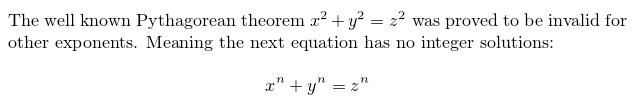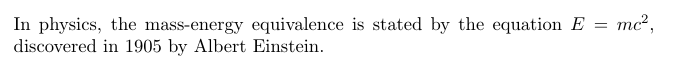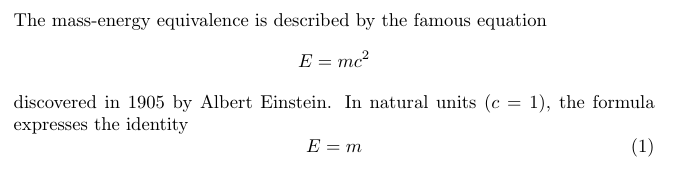Showing first {{hits.length}} results of {{hits_total}} for {{searchQueryText}}{{hits.length}} results for {{searchQueryText}}

Aucun résultat pour la recherche

The feature that makes LaTeX the right editing tool for scientific documents is the ability to render complex mathematical expressions. This article explains the basic commands to display equations. Contents 1 Introduction 2 Mathematical modes 3 Reference guide 4 Further Reading Introduction Basic equations in LaTeX can be easily "programmed", for example: The well known Pythagorean theorem $$x^2 + y^2 = z^2$$ was proved to be invalid for other exponents. Meaning the next equation has no integer solutions: $x^n + y^n = z^n$As you see, the way the equations are displayed depends on the delimiter, in this case  and .   Open an example in Overleaf Mathematical modes LaTeX allows two writing modes for mathematical expressions: the inline mode and the display mode. The first one is used to write formulas that are part of a text. The second one is used to write expressions that are not part of a text or paragraph, and are therefore put on separate lines. Let's see an example of the inline mode: In physics, the mass-energy equivalence is stated by the equation $E=mc^2$, discovered in 1905 by Albert Einstein.To put your equations in inline mode use one of these delimiters: ,  or \begin{math} \end{math}. They all work and the choice is a matter of taste. The displayed mode has two versions: numbered and unnumbered. The mass-energy equivalence is described by the famous equation $E=mc^2$ discovered in 1905 by Albert Einstein. In natural units ($c$ = 1), the formula expresses the identity \begin{equation} E=m \end{equation}To print your equations in display mode use one of these delimiters: , \begin{displaymath} \end{displaymath} or \begin{equation} \end{equation} Important Note: equation* environment is provided by an external package, consult the amsmath article.   Open an example in Overleaf Reference guide Below is a table with some common maths symbols. For a more complete list see the List of Greek letters and math symbols: description code examples Greek letters \alpha \beta \gamma \rho \sigma \delta \epsilon $$\alpha \ \beta \ \gamma \ \rho \ \sigma \ \delta \ \epsilon$$ Binary operators \times \otimes \oplus \cup \cap $\times$$\otimes$$\oplus$$\cup$$\cap$Relation operators < > \subset \supset \subseteq \supseteq $<\ >\subset \ \supset \ \subseteq \ \supseteq$Others \int \oint \sum \prod $\int \ \oint \ \sum \ \prod$Different classes of mathematical symbols are characterized by different formatting (for example, variables are italicized, but operators are not) and different spacing.   Open an example in Overleaf Further Reading The mathematics mode in LaTeX is very flexible and powerful, there is much more that can be done with it: Subscripts and superscripts Brackets and Parentheses Fractions and Binomials Aligning Equations Operators Spacing in math mode Integrals, sums and limits Display style in math mode List of Greek letters and math symbols Mathematical fonts